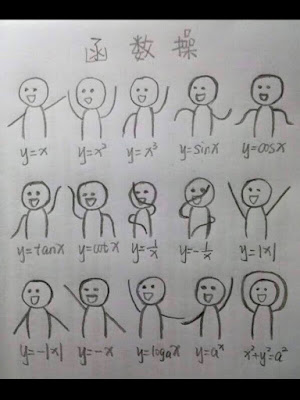## Sunday, March 3, 2019

### Machine Learning Concepts Overview

• Without being explicitly program each step of instruction
• Collect data make observations
• Make use of statistics
• Supervised unsupervised
• Labeled vs unlabeled data
• Features vs labels
• Model: train vs inference
• Regression vs classification
• Bias simplified to 2D is like intercept b in y=wx+b
• We care about minimizing loss across entire dataset.
• SSE will always increase with number of datapoints.
• That's why we like SSE/N averaged out = MSE
• MSE = SSE/n number of data points.
• The MSE isnt always obvious during visual inspection.
• Reducing Loss
• mini-batch gradient descent
• stochastic gradient descent
• Tuning learning rate

Find a direction to go in parameter space to reduce loss.
Compute derivative of loss function --> how to decrease loss.
Take small steps in direction of gradient that min loss
todo derive derivative of MSE
Initialization matters for NN, notoriously non-convex, like an egg carton. Initialization matters more.
Empirically people found there's no need to compute gradient over entire dataset.
Can compute gradient on small data samples
stochastic gradient descent: one example at a time
mini-batch gradient descent: batch of 10-1000
loss & gradient are averaged over the batch
in practice we don't compute the gradient for the entire dataset nor do we compute gradient gradient for one example, instead we do something in the middle: mini batch

We also plan to turn this article into a great ML pattern article on Medium, stay tuned.

Machine learning crash course google